# Counting Polygons in Node Graphs¶

Motivation

• This is what happens when you get curious about efficiently finding triangles in a node graph using linear algebra (instead of recursively walking the whole graph).

Features of custom GraphCounts class:

1. Accepts node/edge data in dictionary format
2. Plots the graph
3. Counts the number of triangles, quadrilaterals, and pentagons.
4. Optionally displays the polygons within the nodes of the graph.
5. Written in python (view source code at bottom).

Author:

# Examples¶

## "House"¶

In :
# Graph Data

# data maps a node to its set of neighbors
# note: if A has neighbor B, then not needed to specify that B has neighbor A
data = {
'A':{'B', 'C'},
'B':{'C', 'D'},
'E':{'C', 'D'}
}

graph = GraphCounts(data, show_matrix=True, show_graph=True)

S1:
[[0. 1. 1. 0. 0.]
[1. 0. 1. 1. 0.]
[1. 1. 0. 0. 1.]
[0. 1. 0. 0. 1.]
[0. 0. 1. 1. 0.]]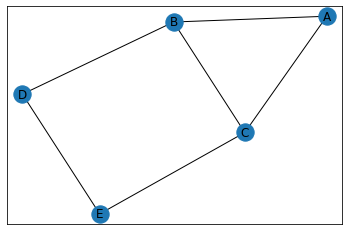In :
triangle_count = graph.count_triangles(show_plots=True)
pentagon_count = graph.count_pentagons(show_plots=True)

print("\n\nCounts")
print("triangles count:", triangle_count)
print("pentagons count:", pentagon_count)

Triangles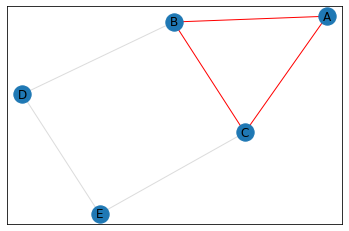Quadrilaterals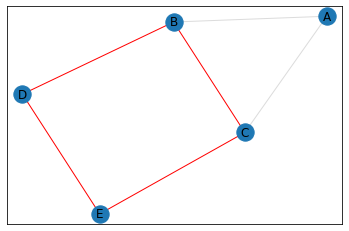Pentagons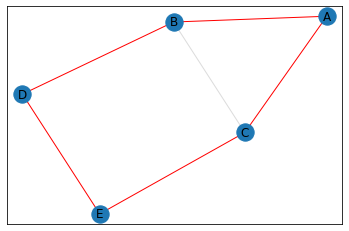Counts
triangles count: 1.0
pentagons count: 1.0


## "Trapezoid"¶

In :
data = {
2:{1,3,4,5},
3:{5},
4:{1,5},
}

graph = GraphCounts(data)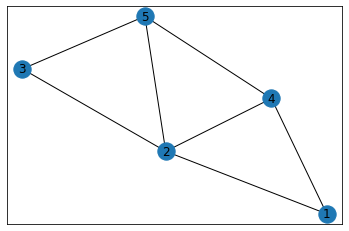In :
triangle_count = graph.count_triangles(show_plots=True)
pentagon_count = graph.count_pentagons(show_plots=True)

print("\n\nCounts")
print("triangles count:", triangle_count)
print("pentagons count:", pentagon_count)

Triangles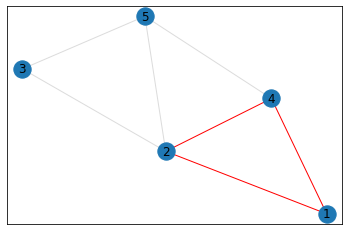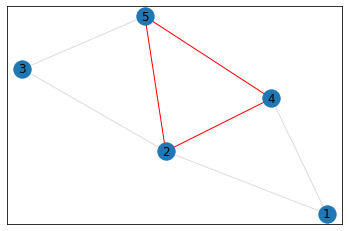Quadrilaterals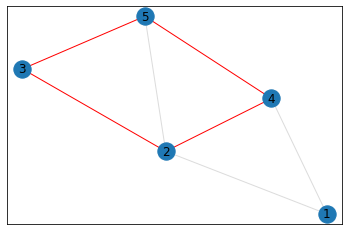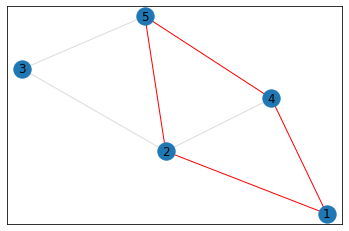Pentagons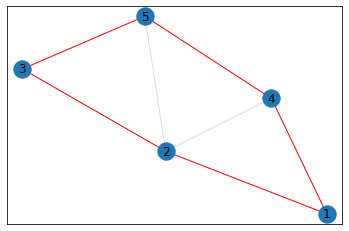Counts
triangles count: 3.0
pentagons count: 1.0


## "Pentagonal Prism"¶

In :
data = {
1:{2,5,6},
2:{3,7},
3:{4,8},
4:{5,9},
5:{10},
6:{7,10},
8:{7,9},
9:{10}
}

graph = GraphCounts(data)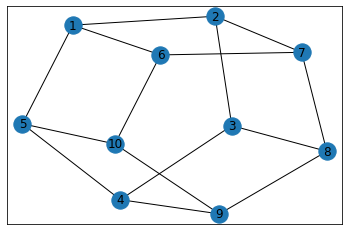In :
triangle_count = graph.count_triangles(show_plots=True)
pentagon_count = graph.count_pentagons(show_plots=True)

print("\n\nCounts")
print("triangles count:", triangle_count)
print("pentagons count:", pentagon_count)

Triangles: none to show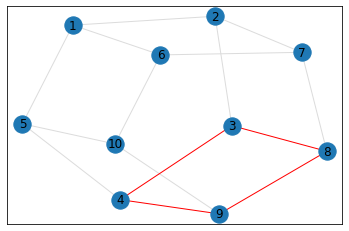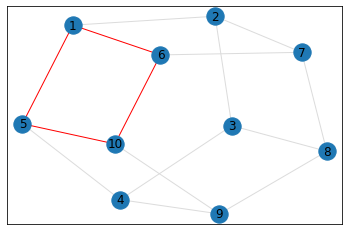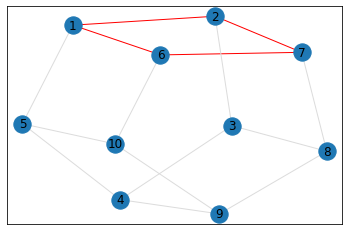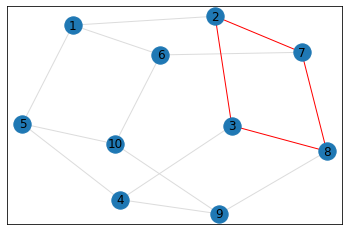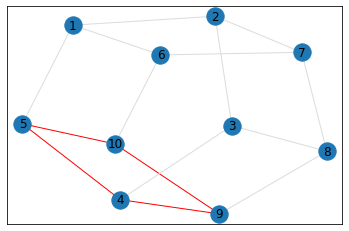Pentagons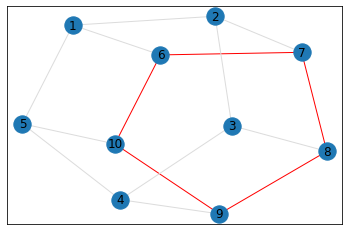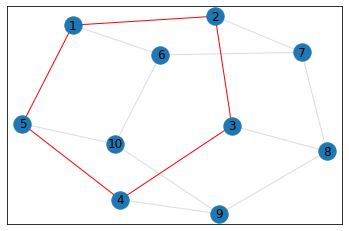Counts
triangles count: 0.0
pentagons count: 2.0


## Random, 15 nodes¶

In :
# generate random graph
data = make_random_graph_data(15, 3)
graph = GraphCounts(data)

Node count: 15, edge_count: 30, avg degree: 2.466666666666667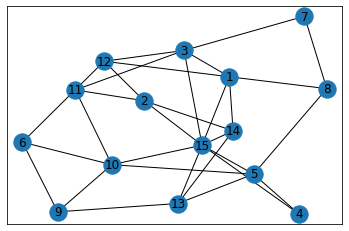In :
triangle_count = graph.count_triangles(show_plots=True)
pentagon_count = graph.count_pentagons(show_plots=True)

print("\n\nCounts")
print("triangles count:", triangle_count)
print("pentagons count:", pentagon_count)

Triangles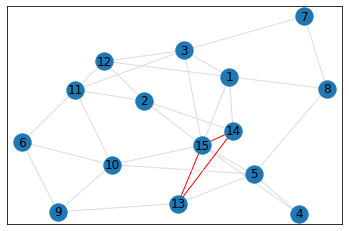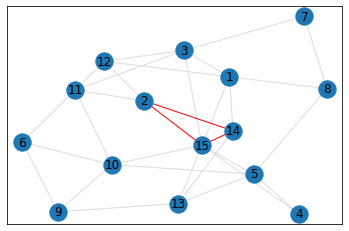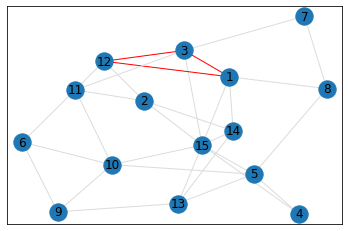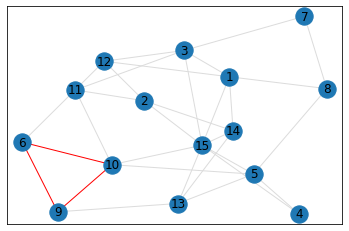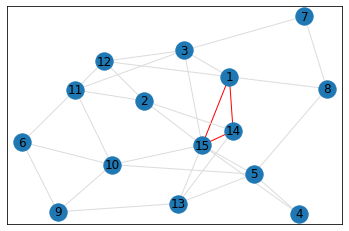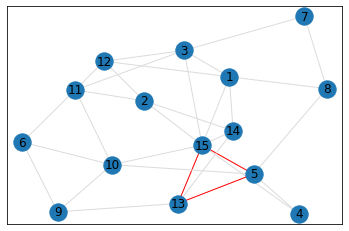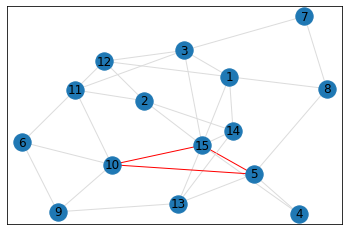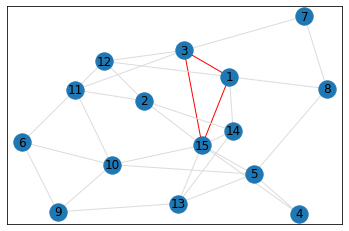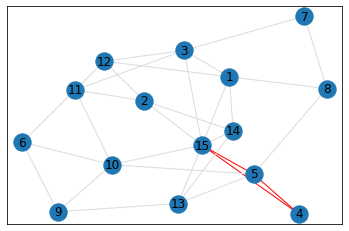Quadrilaterals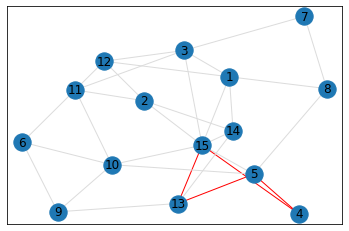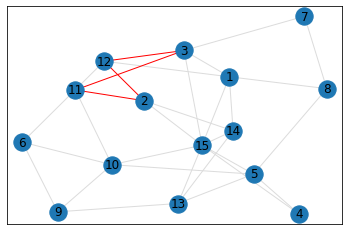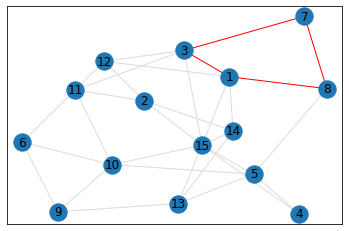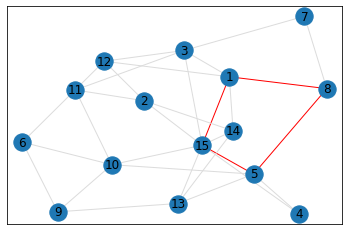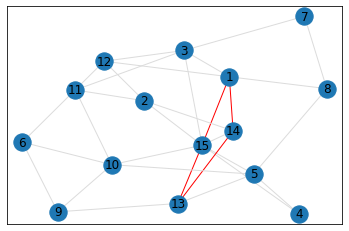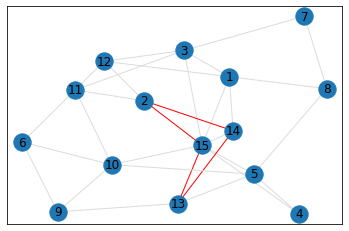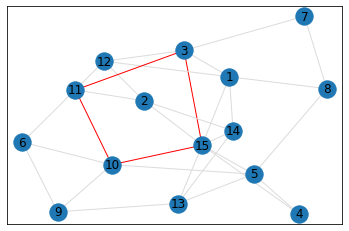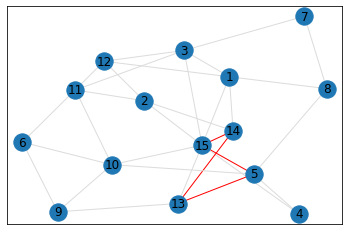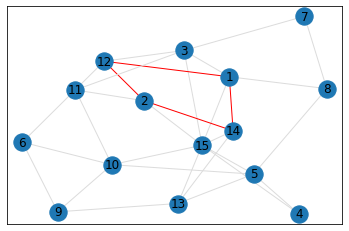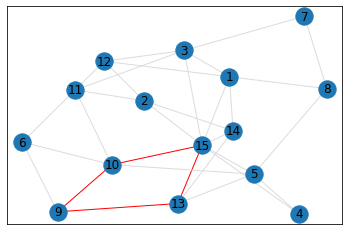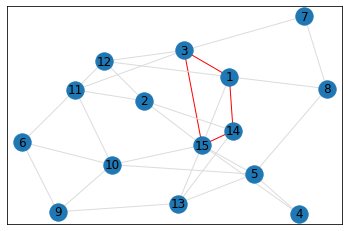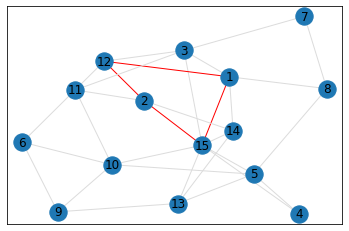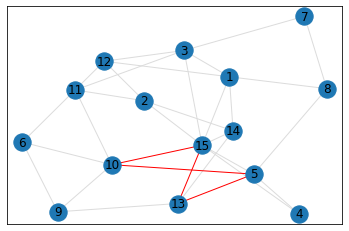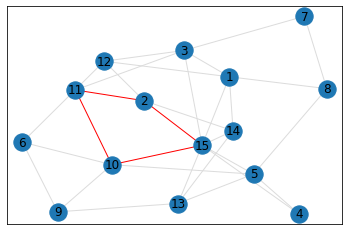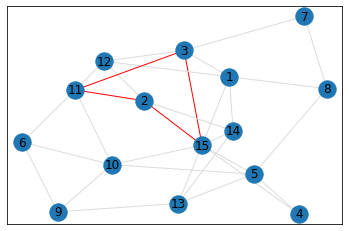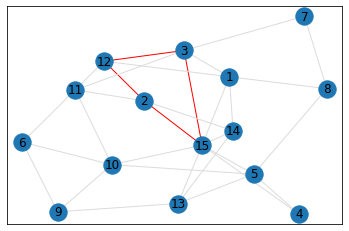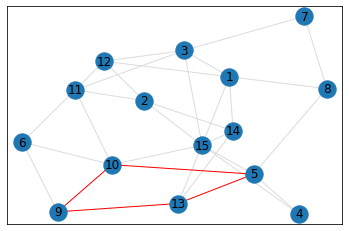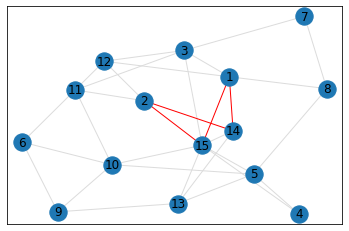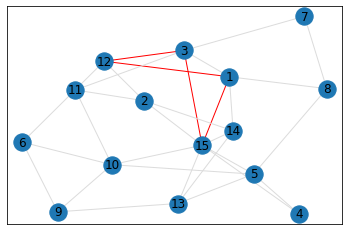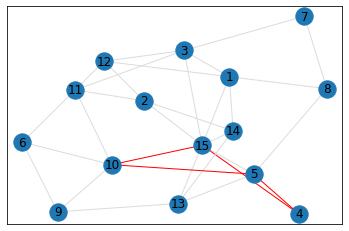Pentagons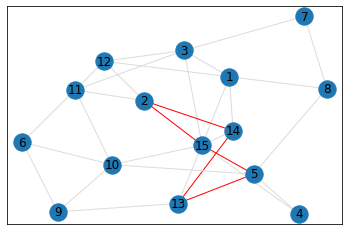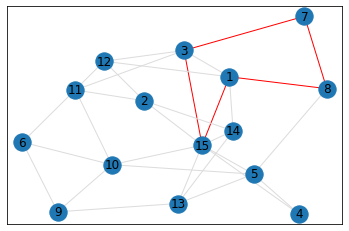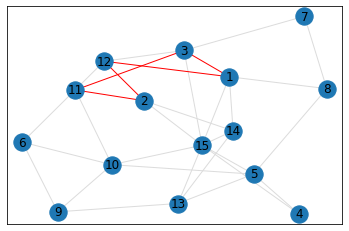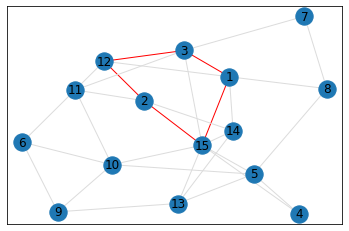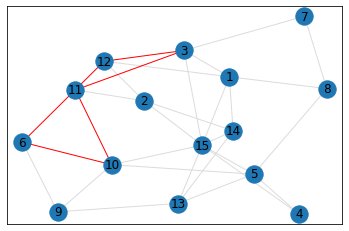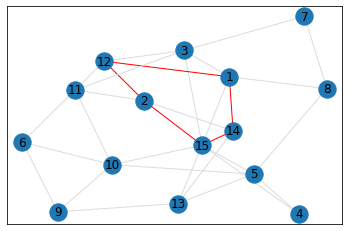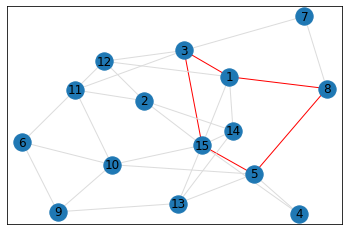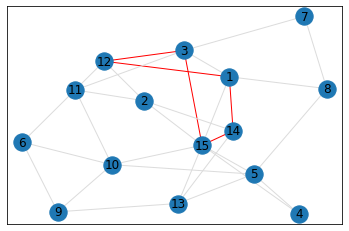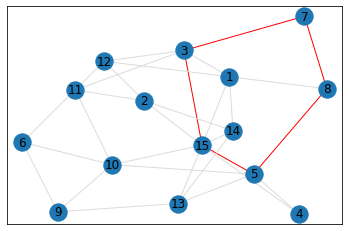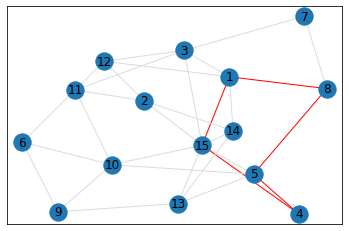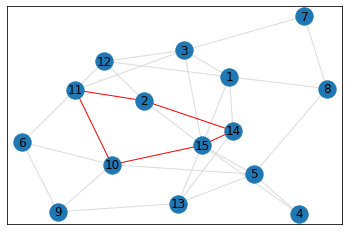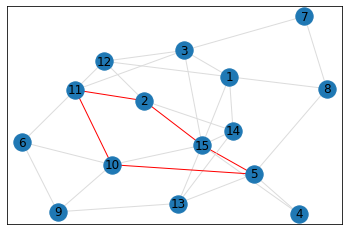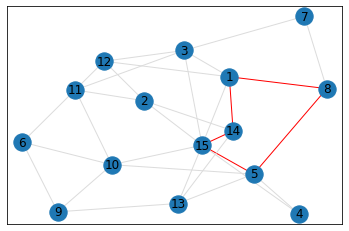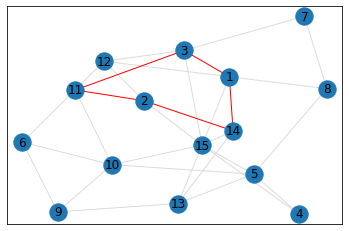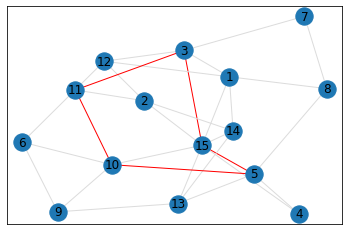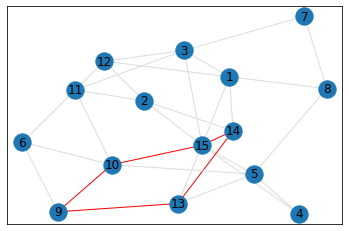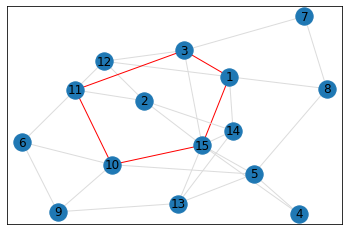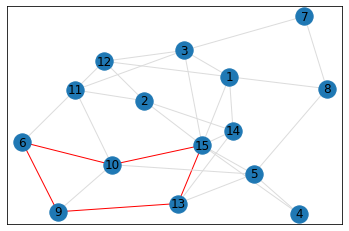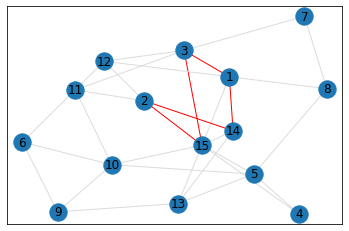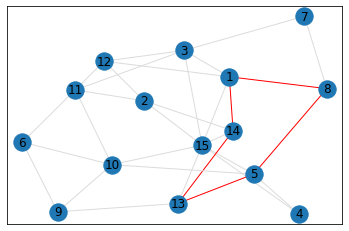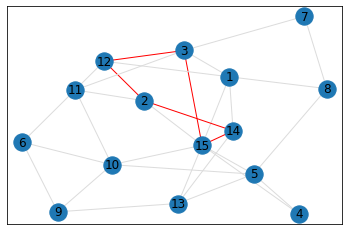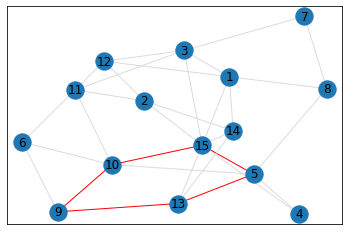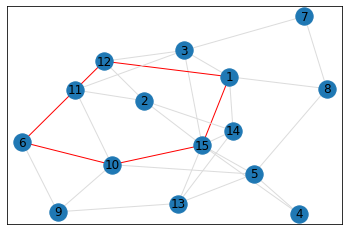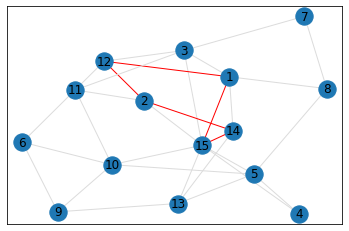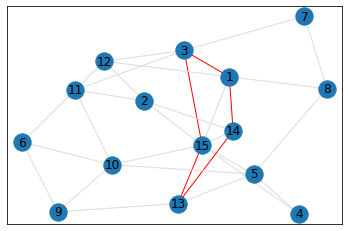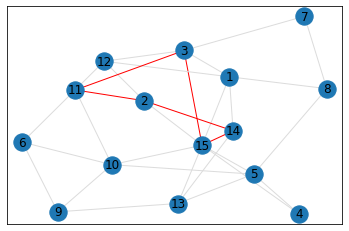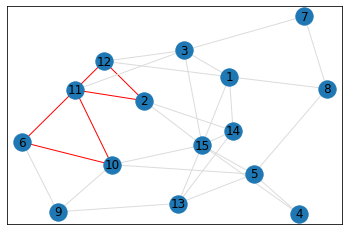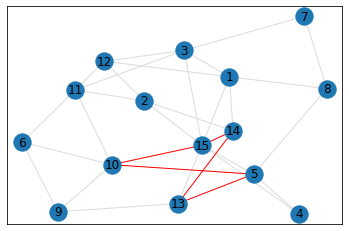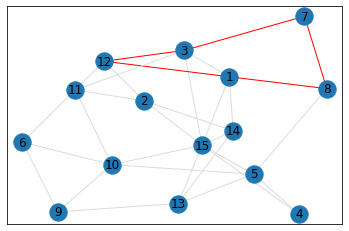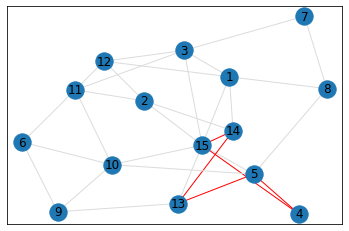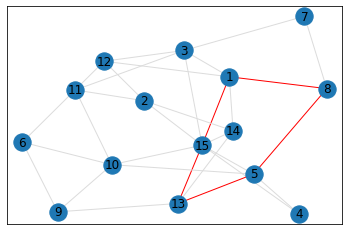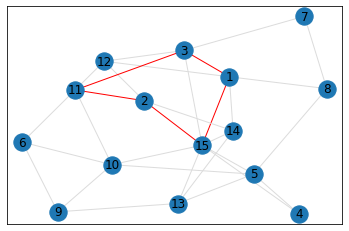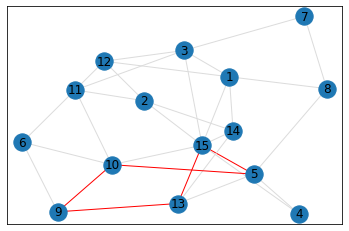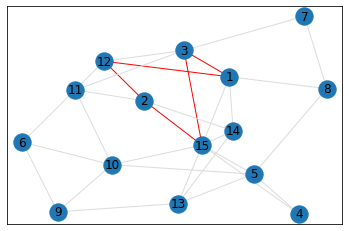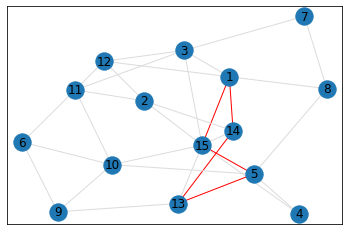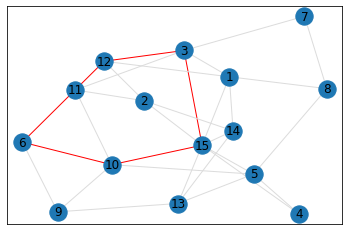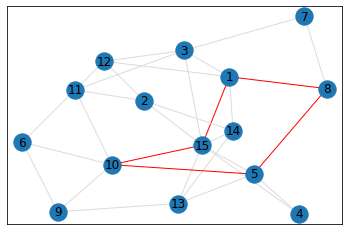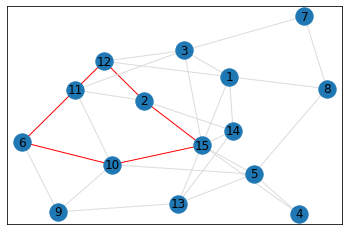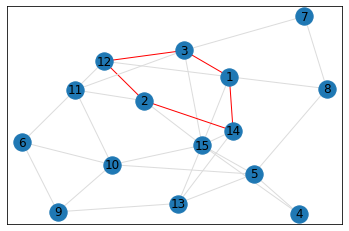Counts
triangles count: 9.0
pentagons count: 40.0


## Random, 1,000 nodes¶

A graph this large needs a much larger display, so graphs will not be shown in this example. But note the efficient execution times.

In :
# generate random graph
data = make_random_graph_data(1000, 3)
graph = GraphCounts(data, show_matrix=False, show_graph=False)

# runtime: 356 milliseconds

Node count: 1000, edge_count: 1955, avg degree: 1.958

In :
print("triangles:", graph.count_triangles())

print("pentagons:", graph.count_pentagons())

# runtime: 256 milliseconds

triangles: 6.0
pentagons: 45.0


## Random, 10,000 nodes¶

A graph this large needs a much larger display, so graphs will not be shown in this example. But note the efficient execution times.

In :
# generate random graph
data = make_random_graph_data(10000, 3)
graph = GraphCounts(data, show_matrix=False, show_graph=False)

# runtime: 21.6 seconds

Node count: 10000, edge_count: 20030, avg degree: 2.0033

In :
graph.count_triangles()

# runtime: 63.0 seconds

Out:
9.0
In :
graph.count_quads()

# runtime: 57.2 seconds

Out:
21.0
In :
graph.count_pentagons()

# runtime: 89.1 seconds

Out:
79.0

## Other Examples¶

### "Kite"¶

In :
data = {
1:{2,3,4},
2:{1,3,4},
3:{1,2,4},
4:{1,2,3},
5:{4,6}
}

graph = GraphCounts(data)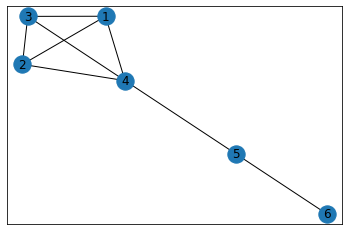In :
print("triangles:", graph.count_triangles())
print("pentagons:", graph.count_pentagons())

triangles: 4.0
pentagons: 0.0


### "Ninja Star"¶

In :
data = {
'A': {'B', 'C', 'D', 'E'},
'F': {'B', 'C', 'E', 'D'},
}

graph = GraphCounts(data)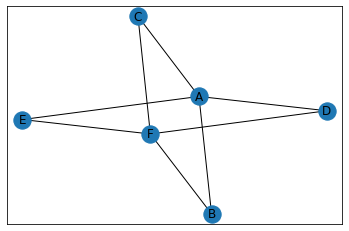In :
print("triangles:", graph.count_triangles())
print("pentagons:", graph.count_pentagons())

triangles: 0.0
pentagons: 0.0


### "Two Windows"¶

In :
data = {
'A':{'B', 'G', 'D', 'H', 'F'},
'B':{'C', 'G'},
'C':{'G'},
'D':{'C', 'G', 'H', 'E'},
'E':{'H'},
'F':{'H', 'E'},
}

graph = GraphCounts(data)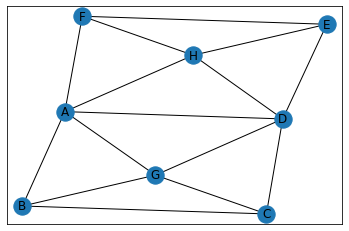In :
print("triangles:", graph.count_triangles())
print("pentagons:", graph.count_pentagons())

triangles: 8.0
pentagons: 14.0


### "Baby Carriage"¶

In :
# maps a node to its set of neighbors
# note: if A has neighbor B, then not needed to specify that B has neighbor A

# rects with triangles
data = {
'A': {'B','C', 'D', 'E', 'F'},
'D': {'B', 'C'},
'G': {'E', 'F'},
'H': {'A', 'C', 'F'},
'E': {'W'}
}

graph = GraphCounts(data)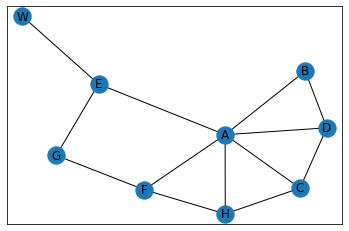In :
print("triangles:", graph.count_triangles())
print("pentagons:", graph.count_pentagons())

triangles: 4.0
pentagons: 3.0


### "Bipolar"¶

In :
data = {
1:{2,3,10},
3:{2,5},
5:{10},
10:{4},
4:{6,7,8},
7:{6,8,9},
8:{9}
}

graph = GraphCounts(data)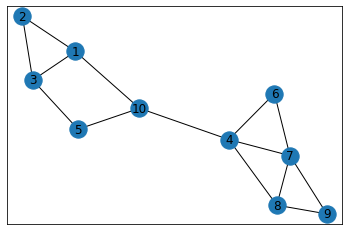In :
print("triangles:", graph.count_triangles())
print("pentagons:", graph.count_pentagons())

triangles: 4.0
pentagons: 2.0


### "Sand Dollar"¶

In :
# star
data = {
1:{2,5,6,7,10},
2:{3,6,7,8},
3:{4,7,8,9},
4:{5,8,9,10},
5:{6,9,10},
6:{7,10},
8:{7,9},
9:{10}
}

graph = GraphCounts(data)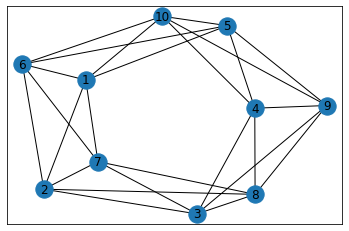In :
print("triangles:", graph.count_triangles())
print("pentagons:", graph.count_pentagons())

triangles: 20.0
pentagons: 72.0


# Source Code¶

Written by Doug Issichopoulos, dougissi.com

Feb 10, 2020

In :
import pandas as pd
import numpy as np
import networkx as nx
from collections import defaultdict
import random
import matplotlib.pyplot as plt

def symmetrify_dict_of_dicts(dict_of_dicts):
sym_dict = defaultdict(dict, dict_of_dicts)
for k, v in dict_of_dicts.items():
for x, y in v.items():
sym_dict[x][k] = y
return dict(sym_dict)

def symmetrify_dict_of_sets(dict_of_sets):
sym_dict = defaultdict(set, dict_of_sets)
for k, v in dict_of_sets.items():
for x in v:
return dict(sym_dict)

def matrix_multiplication(x, y):
return np.matmul(x, y)

def matrix_to_power(S, n):
Sn = S  # initialize output
while n > 1:
Sn = matrix_multiplication(Sn, S)
n -= 1
return Sn

def get_matrix_diagonal_dict(S, nodes):
'''nodes should be ordered the same as the rows/columns of S'''
return {node: val for node, val in zip(nodes, S.diagonal())}

def make_random_graph_data(node_count, max_initial_neighbors):
all_nodes = list(range(1, node_count+1))

data = {}
for node in all_nodes:
all_other_nodes = set(all_nodes).difference({node})
rand_num_neighbors = random.randrange(1,max_initial_neighbors+1)

data[node] = set(random.sample(all_other_nodes, rand_num_neighbors))

# print counts of nodes and edges, as well as avg degree, to console
all_edges = set()
degs = []
for node1, edges_set in data.items():
for node2 in edges_set:
degs.append(len(edges_set))
print(f'Node count: {len(all_nodes)}, edge_count: {len(all_edges)}, avg degree: {sum(degs)/len(degs)}')

return data

class GraphCounts(object):
def __init__(self, data, show_matrix=False, show_graph=True):
'''
data can be dict from node -> {neighbor set}, or node -> {neighbor1: weight, neighbor2: weight,...},
where if B is a neighbor of A, no need to define A as neighbor of B, as weights are ignored.
'''
self.data_dict = symmetrify_dict_of_dicts({k:{x:1 for x in list(v) if x != k} for k, v in data.items()})

# make pandas DataFrame of graph data
df = pd.DataFrame(self.data_dict).fillna(0)
df.sort_index(inplace=True)
df.sort_index(axis=1, inplace=True)
self.df = df

# make matrix
self.S1 = df.values
if show_matrix:
print('S1:\n',self.S1)

# optionally plot graph data using networkx
if show_graph:
self.G, self.pos = self._define_graph()
nx.draw_networkx(self.G, pos=self.pos)
plt.show()

def _define_graph(self):
G = nx.Graph(self.df)
pos = nx.spring_layout(G)
return G, pos

def _get_triangles_dict(self, S3):
returning_3_walks_dict = get_matrix_diagonal_dict(S3, self.df.index)
return {k: v / 2 for k, v in returning_3_walks_dict.items()}

@staticmethod
def _get_nodes_set_with_unidentified_polygons(polygons_dict):
return {node for node, polygon_count in polygons_dict.items() if polygon_count > 0}

@staticmethod
def _polygon_data_to_tuple(data):
'''
data should be dict from node -> {neighbor set},
where if B is a neighbor of A, no need to define A as neighbor of B
'''

sym_dict = symmetrify_dict_of_sets(data)

nodes = set()
edges = set()
for node, edges_set in sym_dict.items():
for edge in edges_set:

return (tuple(sorted(nodes)), tuple(sorted(edges)))

def _identify_polygons(self, polygon_type, polygons_dict):

# helper function
def build_polygons_set(simplified_data_dict, polygon_type, polygons_set, polygons_dict):
'''
Builds polygons_set via recursion, based on number of polygons calculated at each node (from polygons_dict)
'''

for node1, node1_neighbors in simplified_data_dict.items():
for node2 in list(node1_neighbors):
node2_neighbors = simplified_data_dict[node2]

other_node1_neighbors = node1_neighbors.difference({node2})
other_node2_neighbors = node2_neighbors.difference({node1})

if polygon_type == 'triangle':
triangles = set()
common_neighbors = other_node1_neighbors.intersection(other_node2_neighbors)
for node3 in list(common_neighbors):
triangle = self._polygon_data_to_tuple({
node1: {node2, node3},
node2: {node3}
})
if triangle not in polygons_set:
polygons = triangles

connections = set()
for node3 in list(other_node1_neighbors):
for node4 in list(other_node2_neighbors):
if node3 != node4 and node4 in simplified_data_dict[node3]: # nodes different and connection exists between them
connection = tuple(sorted([node3, node4]))
if connection not in connections:  # if new connection then valid polygon
node1: {node2, node3},
node2: {node4},
node3: {node4}
})

elif polygon_type == 'pentagon':
connections = set()
pentagons = set()
for node3 in list(other_node1_neighbors):
for node4 in list(other_node2_neighbors):
if node3 != node4: # nodes different
common_neighbors = set(simplified_data_dict[node3]).intersection(simplified_data_dict[node4]).difference({node1,node2,node3,node4})
for node5 in common_neighbors:
connection = tuple(sorted([node3, node4, node5]))
if connection not in connections:  # if new connection then valid polygon
pentagon = self._polygon_data_to_tuple({
node1: {node2, node3},
node2: {node4},
node3: {node5},
node4: {node5}
})
if pentagon not in polygons_set:
polygons = pentagons

else:
raise ValueError(f"Polygon type '{polygon_type}' not valid.")

if not polygons:
continue

# update storages based on new polygons
for polygon in polygons:

# decrement count of unidentified polygons at node, for each node in polygon
nodes, _ = polygon
for node in nodes:
polygons_dict[node] -= 1

return

## main function

# raise error if invalid polygon type
if polygon_type not in valid_polygon_types:
raise ValueError(f"Polygon type '{polygon_type}' not valid -- must be among: {valid_polygon_types}")

polygons_dict = dict(polygons_dict)  # make copy to avoid mutilation

nodes_set_with_unidentified_polygons = self._get_nodes_set_with_unidentified_polygons(polygons_dict)

simplified_data_dict = {k:{x for x, _ in v.items() if x in nodes_set_with_unidentified_polygons}
for k, v in self.data_dict.items()
if k in nodes_set_with_unidentified_polygons}

polygons_set = set()
while True:
build_polygons_set(simplified_data_dict, polygon_type, polygons_set, polygons_dict)

# limit search based on found polygons above
nodes_set_with_unidentified_polygons = self._get_nodes_set_with_unidentified_polygons(polygons_dict)
if not nodes_set_with_unidentified_polygons:
break

simplified_data_dict = {k:{x for x in v if x in nodes_set_with_unidentified_polygons}
for k, v in simplified_data_dict.items()
if k in nodes_set_with_unidentified_polygons}

return polygons_set

def _plot_polygons(self, polygons_set, desc):

def show_original_graph(G, pos):
print('Original')
nx.draw_networkx(G, pos=pos)
plt.show()

# if graph wasn't originally drawn, define it
if not hasattr(self, 'G') or not hasattr(self, 'pos'):
self.G, self.pos = self._define_graph()
if not polygons_set:  # if no polygons to plot, show original anyway
show_original_graph(self.G, self.pos)

# if no polygons to plot, inform user and return
if not polygons_set:
print(f'{desc}: none to show')
return

all_graph_edges = [tuple(sorted(edge)) for edge in self.G.edges]

# plot each polygon
print(desc)
for _, edges in polygons_set:
polygon_edges = set(edges)
edge_colors = ["red" if edge in polygon_edges else "gainsboro" for edge in all_graph_edges]
nx.draw_networkx(self.G, pos=self.pos, edge_color=edge_colors)
plt.show()

def count_triangles(self, show_plots=False):
S3 = matrix_to_power(self.S1, 3)

if show_plots:
triangles_dict = self._get_triangles_dict(S3)
triangles_set = self._identify_polygons('triangle', triangles_dict)
self._plot_polygons(triangles_set, 'Triangles')

return np.trace(S3) / 6

S2 = matrix_to_power(self.S1, 2)
S4 = matrix_to_power(S2, 2)

degrees_dict = get_matrix_diagonal_dict(S2, self.df.index)
returning_4_walks_dict = get_matrix_diagonal_dict(S4, self.df.index)

# make dict mapping a node to the count of all quadrilaterals passing it
for node in self.data_dict.keys():
quads_dict[node] = 0  # assume none unless proven otherwise

returning_4_walks_at_node = returning_4_walks_dict[node]
if returning_4_walks_at_node < 8:
continue

deg = degrees_dict[node]
if deg < 2:
continue

sum_neighbor_degs = sum([degrees_dict[neighbor] for neighbor in self.data_dict[node]])
if sum_neighbor_degs < 4:
continue

quads_dict[node] = (returning_4_walks_at_node - (deg * (deg - 1) + sum_neighbor_degs)) / 2

if show_plots:

return sum(quads_dict.values()) / 4  # account for double counting for each of quad's 4 nodes

def count_pentagons(self, show_plots=False):
S2 = matrix_to_power(self.S1, 2)
S3 = matrix_multiplication(S2, self.S1)
S5 = matrix_multiplication(S3, S2)

returning_5_walks_dict = get_matrix_diagonal_dict(S5, self.df.index)

# get list of triangles
triangles_dict = self._get_triangles_dict(S3)
triangles = self._identify_polygons('triangle', triangles_dict)

# make map from node to list of triangles at that node
triangles_at_nodes_dict = {node: [] for node in self.data_dict}
for triangle in triangles:
nodes, _ = triangle
for node in nodes:
triangles_at_nodes_dict[node].append(nodes)

pentagons_dict = {}
for node in self.data_dict.keys():
pentagons_dict[node] = 0  # assume none unless proven otherwise

returning_5_walks_dict_at_node = returning_5_walks_dict[node]
if returning_5_walks_dict_at_node < 2:
continue

triangles_at_node = triangles_at_nodes_dict[node]

# walks from triangles at node
walks_from_triangles_at_node = 10 * len(triangles_at_node)

# walks from all edges adjacent to triangles at node
for triangle in triangles_at_node:

# walks from edges from node not part of triangle
tri_neighbors = set(triangle).difference({node})
other_node_neighbors = set(self.data_dict[node]).difference(tri_neighbors)

# walks from edges leaving triangle (not at node)
tri_1, tri_2 = tri_neighbors
other_neighbors_tri_1 = set(self.data_dict[tri_1]).difference({node, tri_2})
other_neighbors_tri_2 = set(self.data_dict[tri_2]).difference({node, tri_1})
+ len(other_neighbors_tri_2))

# walks from single edges connecting node to other triangles
walks_from_single_edges_connecting_node_to_other_triangles = 0
for neighbor in self.data_dict[node]:
disjoint_neighbor_triangles = [tri for tri in triangles_at_nodes_dict[neighbor] if node not in tri]
walks_from_single_edges_connecting_node_to_other_triangles += (2 * len(disjoint_neighbor_triangles))

# formula
pentagons_dict[node] = (returning_5_walks_dict_at_node
- (walks_from_triangles_at_node